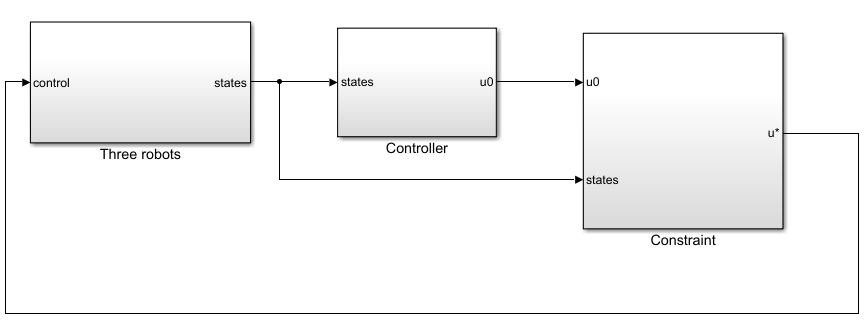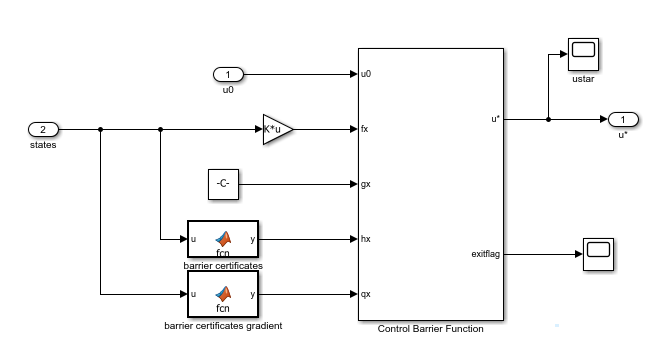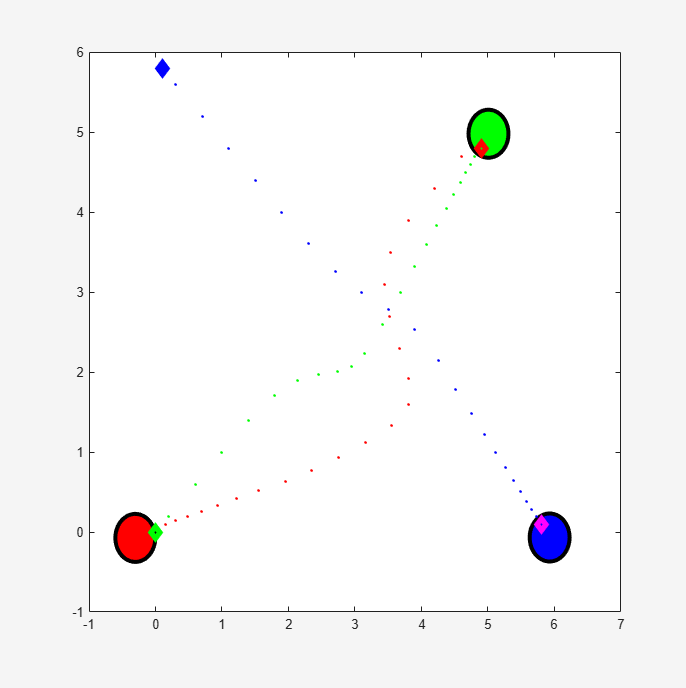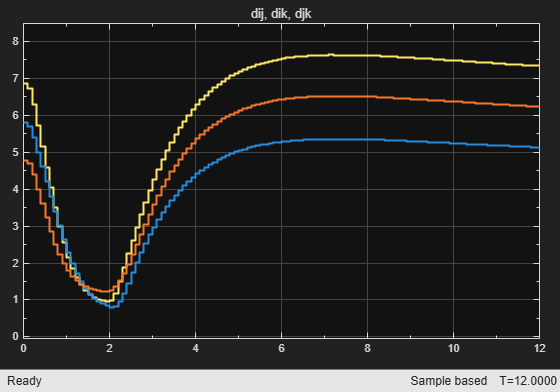# Enforce Barrier Certificate Constraints for Collision-Free Multi-Robot System

This example shows how to enforce barrier certificate constraints for collision-free multi-robot system using the Barrier Certificate Enforcement block.

### Overview

In this example, the goal for the three robots is to reach a target position without colliding with each other . For an example that has two robots, see Enforce Barrier Certificate Constraints for Collision-Free Robots.

The robot dynamics are modeled by double integrators in the x-y plane. Each robot has four states (x-position, y-position, x-velocity, and y-velocity) and two control variables (x-acceleration and y-acceleration). The velocities are constrained to be [-2, 2] m/s and the accelerations are constrained to be [-10, 10] $\mathrm{m}/{\mathrm{s}}^{2}$. The PID controllers are designed for the robots to reach the target position. Before you apply the constraints, the robots collide when they move closer to each other.

```mdl = 'barrierCertificate3Robots'; open_system(mdl);```### Barrier Certificate Constraints

For collision avoidance, the constraint is that the distance between any two robots ($\mathit{i}$, $\mathit{j}$, and $\mathit{k}$) stay greater than a given threshold if the two robots are moving closer to each other . The barrier certificate for robot $\mathit{i}$ and robot $\mathit{j}$ is thus given by

${h}_{ij}\left(x\right)=\sqrt{2{\alpha }_{sum}\left(‖▵{p}_{ij}‖-{D}_{s}\right)}+\frac{▵{p}_{ij}^{T}▵{v}_{ij}}{‖▵{p}_{ij}‖}$.

Here, the variables are:

• The maximum braking power from both robots — ${\alpha }_{\mathrm{sum}}=20$

• The minimum distance between the robots — ${\mathit{D}}_{\mathit{s}}=0.7$

• The position error vector — $▵{p}_{ij}={p}_{i}-{p}_{j}$

• The velocity error vector — $▵{v}_{ij}={v}_{i}-{v}_{j}$

Similarly, the barrier certificate for robot $\mathit{i}$ and robot $\mathit{k}$ is given by ${\mathit{h}}_{\mathrm{ik}}$ and for robot $\mathit{j}$ and robot $\mathit{k}$ is given by ${\mathit{h}}_{\mathrm{jk}}$. The barrier certificates for the multi-robot system are given by $h\left(x\right)=\left[{h}_{ij}\left(x\right),{h}_{ik}\left(x\right),{h}_{jk}\left(x\right){\right]}^{T}$.

The partial derivative of $\mathit{h}\left(\mathit{x}\right)$ over states $\mathit{x}={\left[{\mathit{p}}_{\mathit{i}},{\mathit{p}}_{\mathit{j}},{\mathit{v}}_{\mathit{i}},{\mathit{v}}_{\mathit{j}},{\mathit{p}}_{\mathit{k}},{\mathit{v}}_{\mathit{k}}\right]}^{\mathit{T}}$ is denoted by $\mathit{q}\left(\mathit{x}\right)$.

This example uses Symbolic Math Toolbox™ software to derive the barrier certificates and their gradient functions.

The `getBarrierCertificateAndJacobian` script provided with this example generates the following files:

• `barrierFcn3Robots.m` — Barrier certificates function

• `barrierGradFcn3Robots.m` — Jacobian of barrier certificates

Run the script.

`getBarrierCertificateAndJacobian;`
```ans = function_handle with value: @barrierFcn3Robots ```
```ans = function_handle with value: @barrierGradFcn3Robots ```

For details on either function, open the corresponding file.

The Barrier Certificate Enforcement block accepts the dynamics in the form $\underset{}{\overset{˙}{x}}=f\left(x\right)+g\left(x\right)u$. In this example,

$\mathit{f}\left(\mathit{x}\right)=\left[\begin{array}{cc}0& \mathit{I}\\ 0& 0\end{array}\right]\mathit{x}$ and $\mathit{g}\left(\mathit{x}\right)=\left[\begin{array}{c}0\\ \mathit{I}\end{array}\right]$.

The identity matrix $\mathit{I}$ is a 6-by-6 matrix.

### Simulate Collision-Free Controller with Barrier Certificate Constraint

To view the constraint implementation, open the `Constraint` subsystem.Run the model and view the simulation results. The three robots avoid each other when they are too close.

```mdl = 'barrierCertificate3Robots'; sim(mdl);```Open the scope in the `Three robots` > `visualization` > `distance` subsystem. The distance between any two robots is above the threshold 0.7 m.The Barrier Certificate Enforcement block successfully constrains the control actions such that the three robots reach their target positions in a collision-free manner.

```bdclose(mdl) f = findobj('Name','Three robots'); close(f)```

### References

 Wang, Li, Aaron D. Ames, and Magnus Egerstedt. “Safety Barrier Certificates for Collisions-Free Multirobot Systems.” IEEE Transactions on Robotics 33, no. 3 (June 2017): 661–74. https://doi.org/10.1109/TRO.2017.2659727.